# IV. 2MASS Data Processing

## 5. Extended Source Identification and Photometry

### g. Stellar Number Density and Confusion Noise

As the confusion noise becomes appreciable, it is one of the primary limits on galaxy detection and reliability. Moreover, confusion decreases the accuracy of both flux and position estimation. It is therefore important to understand the confusion noise in terms of the ability to detect isolated sources and in terms of identification of real extended sources, both of which require threshold adjustment with confusion noise level.

To estimate the additional component of "confusion" noise, we adopt the methodology of Hacking (1987, PhDT) and Hacking & Houck (1987, ApJS, 63, 311). The idea is to integrate the expected number of sources (with some flux distribution) within the 2MASS effective beam,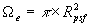, whererepresents the effective radius of the point spread function (typically ~2´´). We may approximate the stellar flux distribution with a power law of index,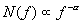(Eq. IV.5.13)

where N is the integrated stellar number density (in deg2) at flux f (in mJy). The aggregate variance due to background sources in the beam, derived fromf, dand the differential stellar number density, is then(Eq. IV.5.14)

where Dc represents the outlier or "deflection" cutoff point (in n-units; i.e., the detection threshold). The source density indexis approximately equal to unity (more precisely ~0.85 for high latitude fields) as derived from the log-log slope of the N vs. Ks cumulative star count curve, ~0.35 for the NGP and slightly steeper at higher densities (Jarrett 1992, PhDT). Letting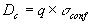, we may express the confusion noise as a function of the stellar number density, N(flim), at the limiting flux, flim, and the deflection cutoff, q,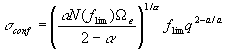(Eq. IV.5.15)

Appropriate values for 2MASS Ks-band data are the following:= 0.9,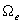= 13.6 arcsec2 (4´´ beam), flim = 1.8 mJy (corresponding to Ks=14 mag), and q between 3 and 5; the confusion noise has units of mJy.

ii. Confusion Noise, Stellar Density and Galactic Coordinates

For relatively moderate flux ranges (e.g., V < 18; Ks < 14), basic three-component models of the Galactic stellar distribution adequately describe the number density of dwarf and giant stars comprising "disk" and "spheroid" populations (Elias 1978, ApJ, 224, 453; Bahcall & Soneira 1980, ApJS, 44, 73; Garwood & Jones 1987, PASP, 99, 453). Here we employ a near-infrared modified variation on the Bahcall & Soneira model, which predicts the stellar number density with ~90% accuracy for most of the sky (|b| > 30°) and Ks < 14 mag, and performs adequately (~80%) for the Galactic plane where patchy extinction ultimately limits the utility of these simple models. The star-count model predicts the stellar number density as a function of the Galactic coordinates, which can then be used to calculate the approximate confusion noise.

A plot of the stellar number density (see IV.5c) as a function of the Galactic latitude along two separate longitudes (50° and 130°) is shown in Figure 30. The vertical dotted lines represent the thresholds for what is deemed low stellar number density (<103.1 stars deg-2), moderate density (<103.6 stars deg-2), and high density (>103.6 stars deg-2). The limit on high density is partly driven by the relative density of triple stars vs. double or single stars. As triple+ stars become appreciable, the ability to distinguish real galaxies from close groupings of stars is greatly diminished. Finally, the confusion noise (mag) appropriate to the stellar number density is plotted in Figure 30 (denoted with a cross-hatching, showing the detection threshold range in q between 3 and 5), with the confusion noise axis located at the right of the plot. Here the confusion noise (in mag units) is relative to the equivalent sky background surface brightness (1-detection limit) measured at high galactic latitudes (i.e., NGP), equal to 0.0016 mJy (in a 4´´ circular diameter beam) or 21.6 mag at 2.2 µm. This relative confusion noise is called the "differential" confusion noise above.

[Last Updated: 2003 Mar 10; by T. Jarrett, T. Chester, S. Schneider, S. Van Dyk, & R. Cutri]